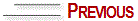# HOW TO DRAW A SPIRAL STAIRCASE IN PARALLEL PERSPECTIVE

 Draw the distance, support and horizon lines and the vanishing, view and depth points inside the visual field, as previously explained. Draw a square corresponding to the diameter of the staircase and divide it into angles of 45º. Find the bisectrix of these 45º angles as follows: Multiply the length of the angle by the expression 0.586. Figure nº 25 illustrates a square of 10x10cm (2 x 2 m in a scale of 1:20). In this case, the length of the angle is 5cm - multiply it by 0.586                                 0.586 x 5 = 2.930                           round up to 3 cmMark 3 cm on the square base (width measurement line) starting at each vertex. See figure nº 25. From this point to the vanishing and depth points, find the lateral 3 cm and so forth. After finding the points on the square edges join these points with the lines that will pass by the perspective center of the square.

Now you have all the angles of the staircase steps already in perspective.

Figure nº 26 shows a circle drawn on the angles of the steps.

Figure nº 27 shows the raising of the steps within a box.

Figure nº 28 shows the spiral staircase already constructed.© Yvonne Tessuto Tavares - All rights reserved